# SAT Math Multiple Choice Question 144: Answer and Explanation

### Test Information

Question: 144

9. If 8x + 8y = 18 and x2 - y2 = -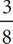, what is the value of 2x - 2y ?

• A. -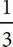• B. -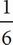• C.• D.A Start by simplifying 8x + 8y = 18 by dividing each term by 8: x + y =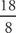or : x + y =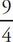. The second equation provided in the question can be factored: x2 - y2 is the same as (x + y)(x - y), so the second equation can also be written (x + y)(x - y) = -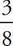. Since you know that x + y =, you can rewrite the second equation as(x - y) = -. Multiply both sides by :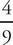: x - y = -() or x - y = -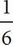. Since the question asks for the value of 2x - 2y, simply multiply everything by 2: 2(x - y) = 2(-) = -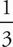, which is (A).